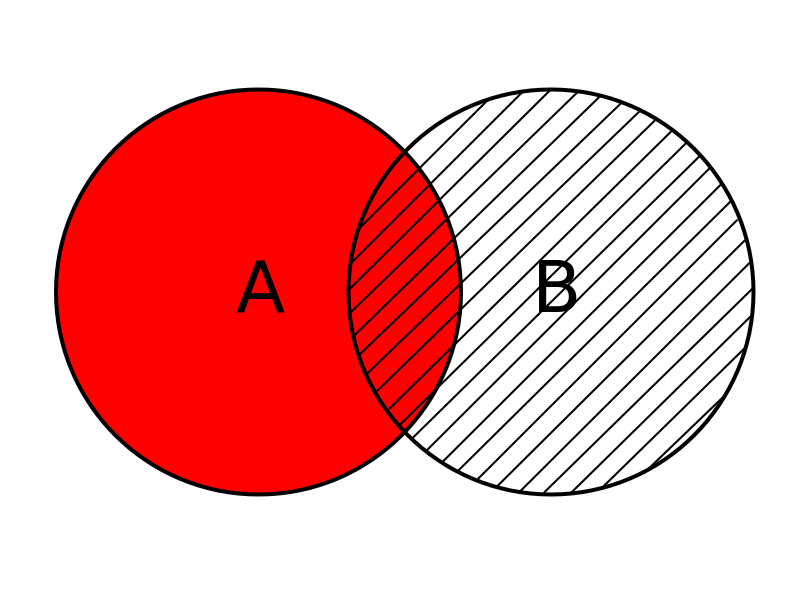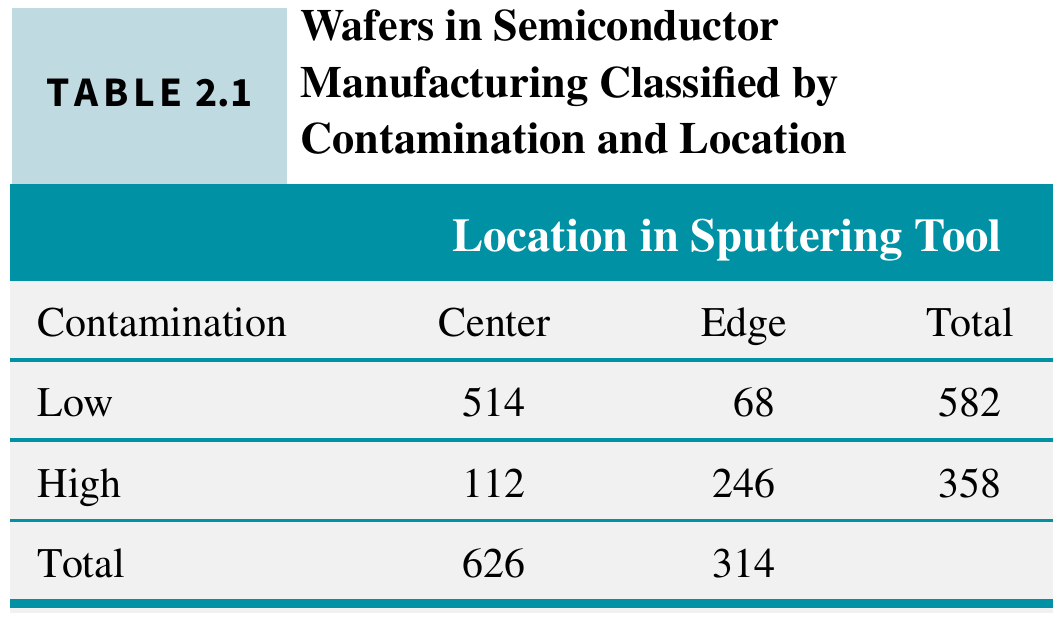# Conditional probability and probability rules

02/03/2021

## Instructions:

Use the left and right arrow keys to navigate the presentation forward and backward respectively. You can also use the arrows at the bottom right of the screen to navigate with a mouse.

FAIR USE ACT DISCLAIMER:
This site is for educational purposes only. This website may contain copyrighted material, the use of which has not been specifically authorized by the copyright holders. The material is made available on this website as a way to advance teaching, and copyright-protected materials are used to the extent necessary to make this class function in a distance learning environment. The Fair Use Copyright Disclaimer is under section 107 of the Copyright Act of 1976, allowance is made for “fair use” for purposes such as criticism, comment, news reporting, teaching, scholarship, education and research.

## Outline

• The following topics will be covered in this lecture:

• Axioms of probabilty
• Conditional probability
• Multiplication rule

## Axioms of Probability

• Now that we are more familiar with the notions of probability, we can collect the assumptions into a set of axioms of probability that must be satisfied in any random experiment.
• Probability is a number that is assigned to each member of a collection of events from a random experiment that satisfies the following properties:
1. $$P(S) = 1$$ where $$S$$ is the sample space
2. $$0 ≤ P(E) ≤ 1$$ for any event $$E$$
3. For two events $$E_1$$ and $$E_2$$ with $$E_1 \cap E_2=\emptyset$$ $P(E_1\cup E_2)=P(E_1)+P(E_2)$
• These axioms do not determine probabilities; the probabilities are assigned based on our knowledge of the system under study.
• These axioms imply some important results
• Probability of the empty set is zero $P(\emptyset)=0$
• We can recognize this as $P(S \cup \emptyset ) = P(S)$ and $P(S \cup \emptyset ) = P(S) + P(\emptyset)$
• Putting the above together, we have $P(S) + P(\emptyset) = P(S) \Leftrightarrow P(\emptyset) = 0.$

### Axioms of Probability – continued

• Probability that event $$E$$ does not occur $P(E')=1-P(E)$
• Notice that $P(E' \cup E) = P(S) = 1$ and $P(E' \cup E) = P(E') + P(E)$
• Therefore, we have $P(E') + P(E) = 1 \Leftrightarrow P(E') = 1 - P(E).$
• If the event $$E_1$$ is contained in the event $$E_2$$, $P(E_1)\leq P(E_2)$
• Notice that $$E_1 \cup E_2 = E_2$$ and $$E_1 \cap E_2 = E_1$$ because of the set containment.
• Therefore, $P(E_2) = P(E_1 \cup E_2 ) = P\left((E_1\cap E_2) \cup (E_1'\cap E_2)\right) = P(E_1)+ P(E_1'\cap E_2)$
• Considering the above, we have $P(E_1) = P(E_2)- P(E_1'\cap E_2)$ where $$P(E_1'\cap E_2) \geq 0$$.

## Unions of Events and Addition RulesCourtesy of Bin im Garten CC via Wikimedia Commons

• More generally, suppose we want to compute the probability of two events $$A$$ and $$B$$ joined by the compound operation “or” that are not disjoint.
• We read the statement, $P(A \text{ or } B)$ as the probability of event:
• $$A$$ occuring,
• event $$B$$ occuring, or
• both $$A$$ and $$B$$ ocurring.
• Intuitively, we can express the probability in terms of all the ways $$A$$ can occur and all the ways $$B$$ can occur, if we don’t double count.
• Consider, if there is an overlap where both $$A$$ and $$B$$ occur simultaneously, $P(A \cap B)\neq \emptyset$ then summing the total of all ways $$A$$ occurs and the total of all ways $$B$$ occurs double counts the the cases where both $$A$$ and $$B$$ occur.
• Therefore, the addition rule for compound events is given as
• Probability of a union $P(A \cup B) = P(A) + P(B) - P(A \cap B)$

## Probability of a union –example

• EXAMPLE: the table below lists the history of 940 wafers in a semiconductor manufacturing process.Courtesy of Montgomery & Runger, Applied Statistics and Probability for Engineers, 7th edition

• Question: whats is the probability that a wafer is from the center of the sputtering tool or contains high levels of contamination ?
• Suppose that 1 wafer is selected at random.
• Total number of outcomes is $$626+314=940$$.
• Let $$H$$ denote the event that the wafer contains high levels of contamination. Then, $P(H) = \frac{358}{940}$
• Let $$C$$ denote the event that the wafer is in the center of a sputtering tool. Then $P(C) = \frac{626}{940}$
• The event $$H \cap C$$ is the event that the wafer is from the center of the sputtering tool and contains high levels of contamination.Then $P(H \cap C)=\frac{112}{940}$

• We can use the addition rule to obtain \begin{align} P(H \cup C) & = P(H)+P(C)-P(H \cap C)\\ & = \frac{358}{940}+\frac{626}{940}-\frac{112}{940}= \frac{872}{940} \end{align}
• ## Two or more events

• More complicated probabilities, such as $$P(A \cup B \cup C)$$, can be determined by repeated use of the addition rule: $P(A \cup B \cup C) = P[(A \cup B) \cup C] = P(A \cup B) + P(C) − P[(A \cup B) \cap C].$
• We can apply the addition rule again on $$P(A \cup B)= P(A)+P(B)-P(A \cap B)$$
• and we can use the distributed rule for set operations on $P[(A \cup B) \cap C]=P[(A\cap C)\cup (B\cap C)]$
• We apply the addition rule on the right-hand side of the expression above $P[(A\cap C)\cup (B\cap C)] = P(A\cap C)+P(B\cap C )-P(A\cap B \cap C)$
• Finally we put everything together \begin{align} P(A \cup B \cup C) & = P(A \cup B) + P(C) − P[(A \cup B) \cap C]\\ & = P(A)+P(B)-P(A \cap B) + P(C) - P(A\cap C) -P(B\cap C )+P(A\cap B \cap C) \end{align}
• If the events are mutually exclusive, the results simplify considerably…